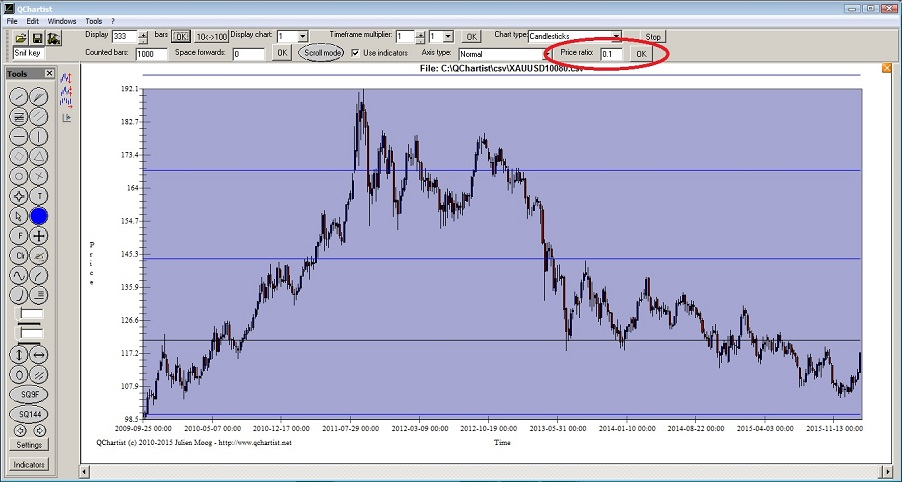Square numbers Previous  Next

Prices naturally gravitate to square numbers ; for example if old high was 122 then 11 squared is 121. (Glen Ring)

If the horizontal lines are too close from each others, you can change the price ratio of the chart. For example if you apply a price ratio of 0.1 this will divide the price by 10 and the distance between the lines will be bigger.